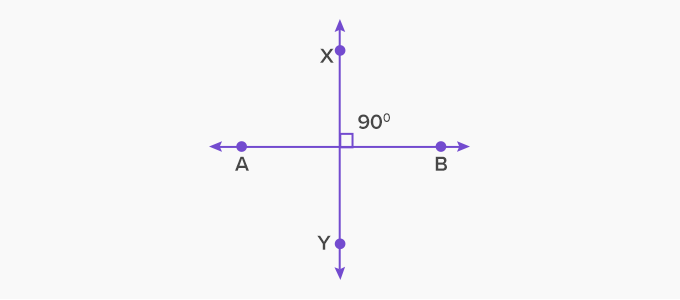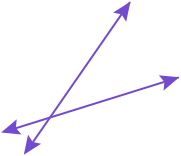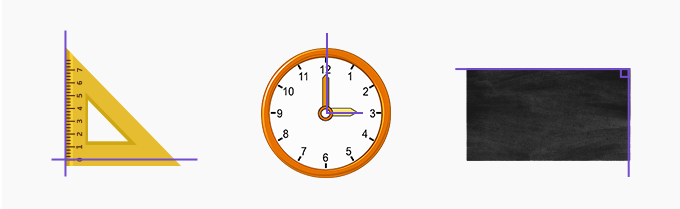# Perpendicular - Definition with Examples

The Complete K-5 Math Learning Program Built for Your Child

• 30 Million Kids

Loved by kids and parent worldwide

• 50,000 Schools

Trusted by teachers across schools

• Comprehensive Curriculum

Aligned to Common Core

What is Perpendicular?

Two distinct lines intersecting each other at 90° or a right angle are called perpendicular lines.

Example:Here, AB is perpendicular to XY because AB and XY intersect each other at 90°.

Non-Example:The two lines are parallel and do not intersect each other. They can never be perpendicular to each other. The two lines are intersecting each other at an acute angle. So, they are not perpendicular. The lines are not  intersecting each other at right angles.

Properties of perpendicular lines

• These lines always intersect at right angles.

• If two lines are perpendicular to the same line, they are parallel to each other and will never intersect.• Adjacent sides of a square and a rectangle are always perpendicular to each other.

• Sides of the right-angled triangle enclosing the right angle are perpendicular to each other.

Real life examples

We can observe many perpendicular lines in real life. Some examples are corner of two walls and the Red Cross symbol.Fun Facts The word ‘perpendicular’ has its origin from the late Middle English which exactly means “at right angles”, from Latin ‘perpendicularis’, ‘perpendiculum’ meaning “plumb line” and from ‘perpendere’ where ‘per’ means “through” and ‘pendere’ means “to hang”. The perpendicular lines are always intersecting lines but intersecting lines are not always perpendicular to each other.

Won Numerous Awards & Honors